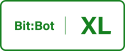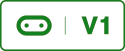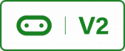# 6. Remote Control RobotTo complete this tutorial you will need another Micro:Bit. In this tutorial we will program another Micro:Bit to control Bit:bot using the radio service.

### Controller

To start we will write the program for the remote controller. The controller will communicate with the robot using the radio service. This utilises the Bluetooth transceiver on the Micro:Bit however it does not use the Bluetooth protocol instead it uses a Nordic propriety protocol.

The controller will send characters to the robot. The controls are as follows;

1. When Button A is pressed, the letter L will be sent to the robot indicating that the robot should turn left.
2. When Button B is pressed, the letter R will be sent to the robot indicating that the robot should turn right.
3. When Button A and button B is pressed, the letter F will be sent to the robot indicating that the robot should go forwards.
4. When no buttons are pressed, the letter S will be sent indicating that the robot should stop.
Remote Controller Code
``````# Controller for the 4tronix Bit:Bot and BBC Micro:Bit

from microbit import *

while True:
txMsg = ""
if button_a.is_pressed() and button_b.is_pressed():
txMsg = "F"
display.show(Image.ARROW_N, loop=False, delay=10)
elif button_a.is_pressed():
txMsg = "L"
display.show(Image.ARROW_W, loop=False, delay=10)
elif button_b.is_pressed():
txMsg = "R"
display.show(Image.ARROW_E, loop=False, delay=10)
else:
txMsg = "S"
display.show(Image.STICKFIGURE, loop=False, delay=10)
sleep(10)``````

I have used a sleep command at the end of the code, this is needed if this is taken out the receiver will receive corrupt data and an error will be displayed. When editing the code keep the delay in or you will have issues.

## Robot

Next we will change the program loop to interpret the commands. The code for the robot can be seen below;

Robot Code
``````# Controlled robot for the 4tronix Bit:Bot and BBC Micro:Bit

from microbit import *

robotType = ""

def detectModel():  # Detects which model were using XL or classic
global robotType
try:
robotType = "XL"  # If we can read it then it must be XL
display.show("X")
except:
robotType = "classic"  # If we can't read it it must be classic
display.show("C")      # or Micro:bit is unplugged
sleep(1000)  # Do this so the user can see if the correct model is found

detectModel()

# Motor pins; these tell the motor to go
# forward, backwards or turn
if robotType == "classic":
leftSpeed = pin0
leftDirection = pin8
rightSpeed = pin1
rightDirection = pin12

else:  # Bit:Bot XL
leftSpeed = pin16
leftDirection = pin8
rightSpeed = pin14
rightDirection = pin12

# Motor control to tell the motor what direction and speed to move
def move(_leftSpeed, _rightSpeed, _leftDirection, _rightDirection):
# speed values between 1 - 1023
# smaller values == faster speed moving backwards
# Smaller values == lower speeds when moving forwards
# direction 0 == forwards, 1 == backwards
leftSpeed.write_analog(_leftSpeed)  # Set the speed of left motor
rightSpeed.write_analog(_rightSpeed)  # Set the speed of right motor
if (_leftDirection != 2):
leftDirection.write_digital(_leftDirection)  # left motor
rightDirection.write_digital(_rightDirection)  # right motor

def drive(speed):
if (speed > 0):
move(speed, speed, 0, 0)  # move the motors forwards
else:
speed = 1023 + speed
move(speed, speed, 1, 1)  # move the motors backwards

def sharpRight():
move(100, 1023 + -200, 0, 1)

def sharpLeft():
move(1023 + -200, 100, 1, 0)

def gentleRight():
move(200, 0, 0, 0)

def gentleLeft():
move(0, 200, 0, 0)

def coast():
move(0, 0, 2, 2)

def stop():
move(0, 0, 0, 0)

while True:
if incoming is not None:
if incoming == "F":
drive(1023)
display.show(Image.ARROW_N, loop=False, delay=10)
elif incoming == "L":
sharpLeft()
display.show(Image.ARROW_W, loop=False, delay=10)
elif incoming == "R":
sharpRight()
display.show(Image.ARROW_E, loop=False, delay=10)
elif incoming == "S":
stop()
display.show(Image.SKULL, loop=False, delay=10)``````

Now upload the Remote code to one Micro:Bit and upload the Robot code to the Micro:Bit that is plugged into Bit:Bot. If you don’t have a battery pack for you remote Micro:Bit you can plug it into you computer via the USB port to power it. Have a play and see how the code works the change the code and add more features. Perhaps you could use the accelerometer or compass to add features.